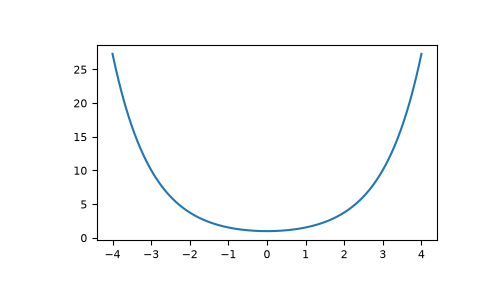# numpy.cosh¶

numpy.cosh(x, /, out=None, *, where=True, casting='same_kind', order='K', dtype=None, subok=True[, signature, extobj]) = <ufunc 'cosh'>

Hyperbolic cosine, element-wise.

Equivalent to `1/2 * (np.exp(x) + np.exp(-x))` and `np.cos(1j*x)`.

Parameters
xarray_like

Input array.

outndarray, None, or tuple of ndarray and None, optional

A location into which the result is stored. If provided, it must have a shape that the inputs broadcast to. If not provided or None, a freshly-allocated array is returned. A tuple (possible only as a keyword argument) must have length equal to the number of outputs.

wherearray_like, optional

This condition is broadcast over the input. At locations where the condition is True, the out array will be set to the ufunc result. Elsewhere, the out array will retain its original value. Note that if an uninitialized out array is created via the default `out=None`, locations within it where the condition is False will remain uninitialized.

**kwargs

For other keyword-only arguments, see the ufunc docs.

Returns
outndarray or scalar

Output array of same shape as x. This is a scalar if x is a scalar.

Examples

```>>> np.cosh(0)
1.0
```

The hyperbolic cosine describes the shape of a hanging cable:

```>>> import matplotlib.pyplot as plt
>>> x = np.linspace(-4, 4, 1000)
>>> plt.plot(x, np.cosh(x))
>>> plt.show()
```